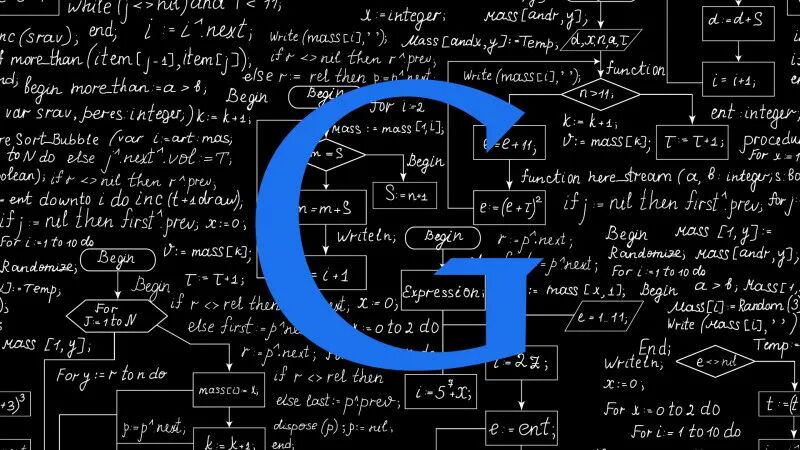# 经典算法设计方法

2023-05-26 0 2431.递推法

【问题】 阶乘计算

N=a[m]×10m-1+a[m-1]×10m-2+ … +a×101+a×100

3 0 2 1 ……

# include <stdio.h>

# include <malloc.h>

# define MAXN 1000

void pnext(int a[ ],int k){

int *b,m=a,i,j,r,carry;

b=(int * ) malloc(sizeof(int)* (m+1));

for ( i=1;i<=m;i++) b[i]=a[i];

for ( j=1;j<=k;j++){

for ( carry=0,i=1;i<=m;i++){

r=(i<a?a[i]+b[i]:a[i])+carry;

a[i]=r%10;

carry=r/10;

}

if (carry) a[++m]=carry;

}

free(b);

a=m;

}

void write(int *a,int k){

int i;

printf(“%4d！=”,k);

for (i=a;i>0;i–)

printf(“%d”,a[i]);

printf(“\n\n”);

}

void main(){

int a[MAXN],n,k;

printf(“Enter the number n: “);

scanf(“%d”,&n);

a=1;

a=1;

write(a,1);

for (k=2;k<=n;k++){

pnext(a,k);

write(a,k);

getchar();

}

}

2.递归

【问题】 编写计算斐波那契（Fibonacci）数列的第n项函数fib（n）。

fib(0)=0;

fib(1)=1;

fib(n)=fib(n-1)+fib(n-2) （当n>1时）。

int fib(int n){

if (n==0) return 0;

if (n==1) return 1;

if (n>1) return fib(n-1)+fib(n-2);

}

【问题】 组合问题

（4）5、3、2 （5）5、3、1 （6）5、2、1

（7）4、3、2 （8）4、3、1 （9）4、2、1

（10）3、2、1

【程序】

# include <stdio.h>

# define MAXN 100

int a[MAXN];

void comb(int m,int k){

int i,j;

for (i=m;i>=k;i–){

a[k]=i;

if (k>1)

comb(i-1,k-1);

else{

for (j=a;j>0;j–)

printf(“%4d”,a[j]);

printf(“\n”);

}

}

}

void main(){

a=3;

comb(5,3);

}

3.回溯法

【问题】 组合问题

（1） a[i+1]>a[i]，后一个数字比前一个大；

（2） a[i]-i<=n-r+1。

【程序】

# define MAXN 100

int a[MAXN];

void comb(int m,int r)

{ int i,j;

i=0;

a[i]=1;

do {

if (a[i]-i<=m-r+1

{ if (i==r-1)

{ for (j=0;j<r;j++)

printf(“%4d”,a[j]);

printf(“\n”);

}

a[i]++;

continue;

}

else

{ if (i==0)

return;

a[–i]++;

}

} while (1)

}

main()

{ comb(5,3);

}

4.贪婪法

【问题】 装箱问题

{ 输入箱子的容积；

for (i=0;i<n;i++)

{ 从已用的第一只箱子开始顺序寻找能放入物品i 的箱子j；

if （已用箱子都不能再放物品i）

{ 另用一个箱子，并将物品i放入该箱子；

box_count++；

}

else

}

}

【程序】

# include <stdio.h>

# include <stdlib.h>

typedef struct ele

{ int vno;

} ELE;

typedef struct hnode

{ int remainder;

Struct hnode *next;

} HNODE;

void main()

{ int n, i, box_count, box_volume, *a;

HNODE *box_h, *box_t, *j;

ELE *p, *q;

Printf(“输入箱子容积\n”);

Scanf(“%d”,&box_volume);

Printf(“输入物品种数\n”);

Scanf(“%d”,&n);

A=(int *)malloc(sizeof(int)*n);

Printf(“请按体积从大到小顺序输入各物品的体积：”);

For (i=0;i<n;i++) scanf(“%d”,a+i);

Box_h=box_t=NULL;

Box_count=0;

For (i=0;i<n;i++)

{ p=(ELE *)malloc(sizeof(ELE));

p->vno=i;

for (j=box_h;j!=NULL;j=j->next)

if (j->remainder>=a[i]) break;

if (j==NULL)

{ j=(HNODE *)malloc(sizeof(HNODE));

j->remainder=box_volume-a[i];

if (box_h==NULL) box_h=box_t=j;

else box_t=boix_t->next=j;

j->next=NULL;

box_count++;

}

else j->remainder-=a[i];

if (q==NULL)

}

else

}

}

printf(“共使用了%d只箱子”，box_count);

printf(“各箱子装物品情况如下：”);

for (j=box_h,i=1;j!=NULL;j=j->next,i++)

{ printf(“第%2d只箱子，还剩余容积%4d，所装物品有；\n”,I,j->remainder);

printf(“%4d”,p->vno+1);

printf(“\n”);

}

}

5.分治法

（1）该问题的规模缩小到一定的程度就可以容易地解决；

（2）该问题可以分解为若干个规模较小的相同问题，即该问题具有最优子结构性质；

（3）利用该问题分解出的子问题的解可以合并为该问题的解；

（4）该问题所分解出的各个子问题是相互独立的，即子问题之间不包含公共的子子问题。

（1）分解：将原问题分解为若干个规模较小，相互独立，与原问题形式相同的子问题；

（2）解决：若子问题规模较小而容易被解决则直接解，否则递归地解各个子问题；

（3）合并：将各个子问题的解合并为原问题的解。

6.动态规划法

【问题】 求两字符序列的最长公共字符子序列

（1） 如果am-1=bn-1，则zk-1=am-1=bn-1，且“z0，z1，…，zk-2”是“a0，a1，…，am-2”和“b0，b1，…，bn-2”的一个最长公共子序列；

（2） 如果am-1!=bn-1，则若zk-1!=am-1，蕴涵“z0，z1，…，zk-1”是“a0，a1，…，am-2”和“b0，b1，…，bn-1”的一个最长公共子序列；

（3） 如果am-1!=bn-1，则若zk-1!=bn-1，蕴涵“z0，z1，…，zk-1”是“a0，a1，…，am-1”和“b0，b1，…，bn-2”的一个最长公共子序列。

# include <stdio.h>

# include <string.h>

# define N 100

char a[N],b[N],str[N];

int lcs_len(char *a, char *b, int c[ ][ N])

{ int m=strlen(a), n=strlen(b), i,j;

for (i=0;i<=m;i++) c[i]=0;

for (i=0;i<=n;i++) c[i]=0;

for (i=1;i<=m;i++)

for (j=1;j<=m;j++)

if (a[i-1]==b[j-1])

c[i][j]=c[i-1][j-1]+1;

else if (c[i-1][j]>=c[i][j-1])

c[i][j]=c[i-1][j];

else

c[i][j]=c[i][j-1];

return c[m][n];

}

char *buile_lcs(char s[ ],char *a, char *b)

{ int k, i=strlen(a), j=strlen(b);

k=lcs_len(a,b,c);

s[k]=’\0’;

while (k>0)

if (c[i][j]==c[i-1][j]) i–;

else if (c[i][j]==c[i][j-1]) j–;

else { s[–k]=a[i-1];

i–; j–;

}

return s;

}

void main()

{ printf (“Enter two string（<%d）!\n”,N);

scanf(“%s%s”,a,b);

printf(“LCS=%s\n”,build_lcs(str,a,b));

}

7.迭代法

（1） 选一个方程的近似根，赋给变量x0；

（2） 将x0的值保存于变量x1，然后计算g(x1)，并将结果存于变量x0；

（3） 当x0与x1的差的绝对值还小于指定的精度要求时，重复步骤（2）的计算。

【算法】迭代法求方程组的根

{ for (i=0;i<n;i++)

x[i]=初始近似根;

do {

for (i=0;i<n;i++)

y[i] = x[i];

for (i=0;i<n;i++)

x[i] = gi(X);

for (delta=0.0,i=0;i<n;i++)

if (fabs(y[i]-x[i])>delta) delta=fabs(y[i]-x[i])；

} while (delta>Epsilon)；

for (i=0;i<n;i++)

printf(“变量x[%d]的近似根是 %f”，I，x[i])；

printf(“\n”)；

} 具体使用迭代法求根时应注意以下两种可能发生的情况：

（1）如果方程无解，算法求出的近似根序列就不会收敛，迭代过程会变成死循环，因此在使用迭代算法前应先考察方程是否有解，并在程序中对迭代的次数给予限制；

（2）方程虽然有解，但迭代公式选择不当，或迭代的初始近似根选择不合理，也会导致迭代失败。

8.穷举搜索法

【问题】 将A、B、C、D、E、F这六个变量排成如图所示的三角形，这六个变量分别取[1，6]上的整数，且均不相同。求使三角形三条边上的变量之和相等的全部解。如图就是一个解。

# include <stdio.h>

void main(){ i

nt a,b,c,d,e,f;

for (a=1;a<=6;a++) {

for (b=1;b<=6;b++) {

if (b==a) continue;

for (c=1;c<=6;c++) {

if (c==a)||(c==b) continue;

for (d=1;d<=6;d++) {

if (d==a)||(d==b)||(d==c) continue;

for (e=1;e<=6;e++) {

if (e==a)||(e==b)||(e==c)||(e==d) continue;

f = 21-(a+b+c+d+e);

if ((a+b+c==c+d+e))&&(a+b+c==e+f+a)) {

printf(“%6d,a);

printf(“%4d%4d”,b,f);

printf(“%2d%4d%4d”,c,d,e);

scanf(“%*c”);

}

}

}

}

}

}

}3.国防科技大学教授：殷建平——计算机科学理论的过去、现在与未来

8.数学的深

10.在美国7年求学执教的见闻和感

11.高考大数据：哪个省才是高考地狱模式？结论和想象不太一样### 相关文章3个月前 6763个月前 6713个月前 1,1313个月前 904